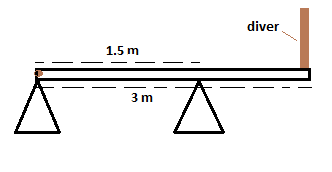# A 57 kg diver stands at the end of a 31 kg springboard as shown. The board is attached to a hinge...

## Question:

A 57 kg diver stands at the end of a 31 kg springboard as shown. The board is attached to a hinge at the left end but simply rests on the right support. What is the magnitude of the vertical force exerted by the hinge on the board?## Equilibrium of Moments of the Force or Torque

Moment of the force or torque is the product of force and perpendicular distance between the point of application of the force and the pivot point. In order to balance clockwise torque we need to apply equal magnitude counter clockwise torque or moment of the force. The effect of torque or moment of the force is rotation about the pivot point.

Given points

• Mass of the diver M = 57 kg
• Length of the spring board L = 3 m
• Distance from the hinge to the right support a = L/2 = 1.5 m
• Mass of the uniform board m = 31 kg
• Acceleration due to gravity {eq}g = 9.8 \ \ m/s^2 {/eq}

The left side is hinged and the right support is exactly at the center of gravity of the board. So in the absence of the diver, the board is balanced and the hinge does not need to exert any force.

When the diver stands at at extreme right end there is a clockwise moment acting on the board about the pivot point.

So in order to balance the board the hinge has to exert a vertical force downwards such that it produces an anti clockwise moment equal in magnitude to the clockwise moment.

Let {eq}F_v {/eq} be the vertical force exerted by the hinge.

Then the equilibrium condition {eq}F_v \times 1.5 = M g \times 1.5 {/eq}

So the required vertical force downwards {eq}F_v = \dfrac { M g \times 1.5 } { 1.5 } \\ = 57 \times 9.8 \\ = 558.60 \ \ N {/eq}